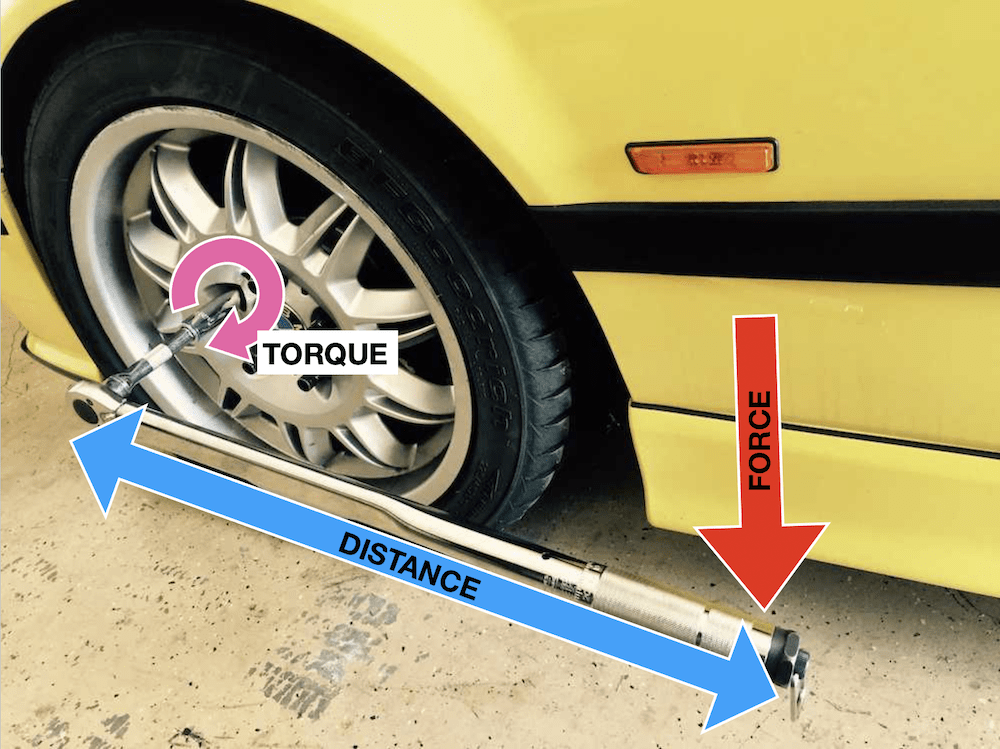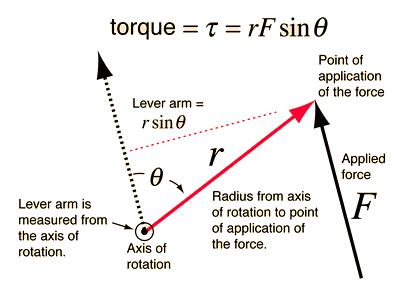# Torque Study Guide

INTRODUCTION

POV: You’re helping your dad change the tires on his car for the season. You pick up a socket wrench from the garage to loosen the fasteners on the hub, but it seems impossible no matter how hard you push on it. Your dad hands your kid brother a power bar, and now he’s breaking those nuts loose like a champ! 😀

What happened? How can he loosen those nuts without breaking a sweat using a longer bar? The answer is torque! This is an incredibly useful physics concept that you can apply in real life, so make sure to know it well.Source

### WHAT IS TORQUE?

Torque (τ) is a twisting or turning force that causes rotation about an axis. Every time you open a jar by turning the lid loose or turning a nut loose with a wrench, you apply a torsion force. Unlike linear force, which causes motion in a straight line, torque causes the rotational motion of objects about an axis of rotation.

In the case of the car tires, you are applying a turning force on the nut by using a socket wrench. Once the nut is sufficiently loose, you can use your fingers to unscrew it just the jar’s lid using less torque.

### THE TORQUE EQUATION

The torque definition mathematically is as simple as the force (F) applied multiplied by the linear distance (r) to the axis of rotation: τ = Fr. In reality, the torque formula is a bit more complicated than that. Consider the figure below:Source

The lever arm, which generates a torque about the axis of rotation, is not always at the same angle relative to the direction of the applied force. Since only the perpendicular component of the force is useful for generating the torque, the force will have to be written as F sinθ in the torque equation.

τ = Fr which gives the final torque formula as:

``                       torque= τ=rFsinθ``

### APPLICATIONS OF TORQUE:

Torsion force is used for different types of applications mentioned below:

• Wrenches and screwdrivers
• Pedalling a bicycle
• Pushing a door open
• The steering wheel of an automobile

## CONCLUSION:

• Torque (τ) is a twisting or turning force that causes rotation about an axis.
• When force remains constant, torque can be multiplied by increasing the length of the lever arm.
• Torque is used in different machines and mechanisms.

### FAQs:

1. How do you define torque?

The torque physics definition explains torque as a turning or twisting force that causes rotation about an axis.

2. How do I calculate torque?

The torque (τ) is a product of the applied force and the distance of the applied force from the axis of rotation. Torque= τ=rFsinθ. In the torque equation, r is the distance to the rotational axis, and Fsinθ is the perpendicular component of the applied force.

3. What are torque and speed?

The torque produced by a motor or engine is the rotational force it can generate. The unit of torqueis Newton-meters (N-m). Speed is the rate at which the motor/engine rotates. It is measured in rotation per minute (rpm).

4. What is torque vs. power?

Power is the total mechanical output of a motor/engine. It is a product of speed and torque. It is measured in Watts (W) or horsepower (hp).

We hope you enjoyed studying this lesson and learned something cool about Torque! Join our Discord community to get any questions you may have answered and to engage with other students just like you! We promise, it makes studying much more fun! 😎

## SOURCES:

1. 8.1 Torque. https://flexbooks.ck12.org/cbook/ck-12-physics-flexbook-2.0/section/8.1/primary/lesson/torque-physics-intermediate/. Accessed 25 jan 2022.
2. Introduction To Torque And Its Applications. https://byjus.com/physics/torque/. Accessed 25 jan 2022.
3. Torque Calculation. http://hyperphysics.phy-astr.gsu.edu/hbase/torq2.html. Accessed 25 jan 2022.
]]>